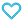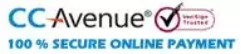# JEE Mains Challenging Mathematics

350.00

JEE Mains Challenging Mathematics book consists of 1100+ Topic wise MCQs that are useful for JEE main/ State Level exams / PUCs. It contains pointwise theory and formulae for last minute revision. It enhances the problem-solving approach of students.

971 in stock

Qty:Add to WishlistJEE Mains Challenging Mathematics book consists of 1100+ Topic wise MCQs that are useful for JEE main/ State Level exams / PUCs. It contains pointwise theory and formulae for last minute revision. It enhances the problem-solving approach of students.

Table of Content:
• Complex Numbers
• Theory of Equations
• Progressions
• Logarithms
• Permutations and Combinations
• Binomial Theorem
• Exponential and Logarithmic Series
• Matrices and Determinants
• Probability
• Trigonometry
• General Solutions to Trigonometric Equations
• Inverse Trigonometric Functions
• Solution of Triangles
• Sets, Relations and Functions
• Limits
• Method of Differentiation
• Applications of Derivatives
• Indefinite Integration
• Definite Integration
• Area Under the Curves
• Differential Equations
• Straight Lines
• Circles
• Conic Section
• Vectors and 3D Geometry
• Basics of Mathematics
ISBN11 9789384248390 Anil Kr. Gupta 2014 344 Class 11, Class 12 JEE Mains-Advanced Mathematics 460gm

## Reviews

There are no reviews yet.